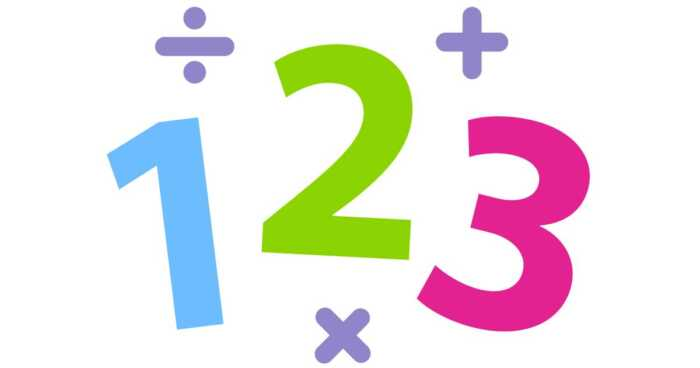Wednesday , December 8 2021

# How to Find the Greatest Common Factors of Two Numbers?Learning to identify the greatest common factors of two numbers is a key math skill highly crucial for calculations particularly in maths. It is quite useful especially for simplifying fractions. Since determining the common denominators for two fractional numbers may seem difficult for students, learning two simple methods for determining the greatest common factor will enable them to understand and learn the process quickly. Although, they need to first have a clear understanding of the basics of factors to learn these two approaches of finding common factors. Eventually, they can apply their acquired knowledge to simplify fractions.

## What Is a Factor?

The factors are numbers multiplied together to give other numbers. For instance, four and two are the factors of eight, because four multiplied by two equals eight. Similarly, three and three are factors of nine, since three multiplied by three is equal to nine. As you may know, prime numbers are numbers that have no factors other than themselves, and one. So 3 is a prime number because the only two whole numbers (integers) that can multiply together to give 3 as an answer are 3 and 1. In the same way, 7 is a prime number, and so is 13.

Because of this, it’s often helpful to break down a number into “prime factors.” This means finding all of the prime number factors of another number. It basically breaks the number down into its fundamental “building blocks,” which is a useful step towards finding the greatest common factor of two numbers and is also invaluable when it comes to simplifying square roots.

## Finding the Greatest Common Factor:

In algebra, the greatest common factor (GCF) of a set of numbers is the largest factor that all the numbers have the same in a given set. This factor should divide all the numbers without any remainder.

There are various methods involved in finding the GCF of a set of given numbers:

• Listing the factors Method.
• Prime Factorization Method.
• Division Method

### Listing the factors Method:

The simplest method for finding the greatest common factor of two numbers is to simply list all of the factors of each number and look for the highest number that both of them share.

This method of finding GCF includes listing all the factors of any given number and then finding the highest common factor among them.

Steps of listing the factors method:

• Write a list of all the factors of each number.
• Mark all the common factors.
• Find the common factor with the greatest value.

### Prime Factorization Method:

This process of determining GCF includes factorization of given numbers into their factors. It is also known as the highest common factor(HCF) or highest common divisor(HCD).  Understanding the concept of GCF requires the knowledge of factors and common factors of numbers.

The prime factorization method includes representing a number as a product of all its prime factors, starting from that number’s smallest prime factor. Steps of prime factorization method:

• Write all the prime factors of any given set of numbers.
• Mark all the common factors among the factors of numbers.
• Multiply the common factors found in each number, and this number is the greatest common factor of the set of numbers.

### Conclusion

Learning the process of finding HCF and LCM is highly important for every student as it forms the basis for understanding many advanced skills. Cuemath enables students to visualize math thus helping them gain a deep understanding of math topics. By gaining a basic understanding of these core concepts students can establish the right approach for learning advanced math with ease.